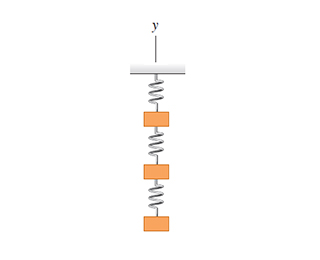# Problem: Three identical 8.50-kg masses are hung by three identical springs . Each spring has a force constant of 7.60 kN/m and was 14.0 cm long before any masses were attached to it.How long is the bottom spring when hanging as shown? (Hint: isolate only the bottom mass.)How long is the middle spring when hanging as shown? (Hint: treat the bottom two masses as a system.)How long is the top spring when hanging as shown? (Hint: treat all three masses as a system.)Draw a free-body diagram for the top mass.Draw a free-body diagram for the middle mass.Draw a free-body diagram for the bottom mass.

⚠️Our tutors found the solution shown to be helpful for the problem you're searching for. We don't have the exact solution yet.

###### Problem Details

Three identical 8.50-kg masses are hung by three identical springs. Each spring has a force constant of 7.60 kN/m and was 14.0 cm long before any masses were attached to it.

How long is the bottom spring when hanging as shown? (Hint: isolate only the bottom mass.)

How long is the middle spring when hanging as shown? (Hint: treat the bottom two masses as a system.)

How long is the top spring when hanging as shown? (Hint: treat all three masses as a system.)

Draw a free-body diagram for the top mass.

Draw a free-body diagram for the middle mass.

Draw a free-body diagram for the bottom mass.